April 2014

Volume 29 Number 4

# DirectX Factor : 3D Transforms on 2D Bitmaps

Charles Petzold | April 2014Three-dimensional graphics programming is mostly a matter of creating optical illusions. Images are rendered on a flat screen consisting of a two-dimensional array of pixels, but these objects must appear to have a third dimension with depth.

Probably the biggest contributor to the illusion of 3D is shading—the art and science of coloring pixels so that surfaces resemble real-world textures with lighting and shadows.

Underneath all that, however, is an infrastructure of virtual objects described by 3D coordinates.  Ultimately, these 3D coordinates are flattened into a 2D space, but until that final step, 3D coordinates are often systematically modified in various ways through the use of transforms. Mathematically, transforms are operations in matrix algebra. Achieving a fluency in these 3D transforms is crucial for anyone who wants to become a 3D graphics programmer.

Recently, I’ve been exploring the several ways 3D is supported in the Direct2D component of DirectX. Exploring 3D within the relative familiarity and comfort of Direct2D allows you to become acquainted with 3D concepts prior to the very scary deep plunge into Direct3D.

## Bitmaps in 3D?

One of the several ways 3D is supported in Direct2D is tucked away as the last argument to the DrawBitmap methods defined by ID2D1DeviceContext. This argument lets you apply a 3D transform to a 2D bitmap. (This feature is special to ID2D1DeviceContext. It is not supported by the DrawBitmap methods defined by ID2D1RenderTarget or the other interfaces that derive from that.)

The DrawBitmap methods defined by ID2D1DeviceContext have a final argument of type D2D1_MATRIX_4X4_F, which is a 4×4 transform matrix that performs a 3D transform on the bitmap as it’s rendered to the screen:

``````void DrawBitmap(ID2D1Bitmap *bitmap,
D2D1_RECT_F *destinationRectangle,
FLOAT opacity,
D2D1_INTERPOLATION_MODE interpolationMode,
const D2D1_RECT_F *sourceRectangle,
const D2D1_MATRIX_4X4_F *perspectiveTransform)
``````

This appears to be sole purpose of D2D1_MATRIX_4X4_F. It isn’t used elsewhere in DirectX. In Direct3D programming, the DirectX Math library is used instead to represent 3D transforms.

DD2D1_MATRIX_4X4_F is a typedef for D2D_MATRIX_4X4_F, which is defined in Figure 1. It’s basically a collection of 16 float values arranged in four rows of four columns. You can reference the value in the third row and second column using the data member _32, or you can get at the same value as the zero-based array element m.

Figure 1 The 3D Transform Matrix Applied to Bitmaps

``````typedef struct D2D_MATRIX_4X4_F
{
union
{
struct
{
FLOAT _11, _12, _13, _14;
FLOAT _21, _22, _23, _24;
FLOAT _31, _32, _33, _34;
FLOAT _41, _42, _43, _44;
} ;
FLOAT m;
};
} D2D_MATRIX_4X4_F;
``````

However, when showing you the mathematics of the transform, I’ll instead refer to the element in the third row and second column of the matrix as m32. The entire matrix can be represented in traditional matrix notation, like so: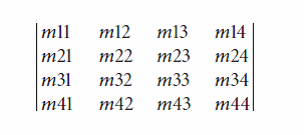The 3D bitmap transform in Direct2D underlies a similar facility in the Windows Runtime, where it shows up as the Matrix3D structure and the Projection property defined by UIElement. The two mechanisms are so similar that you can cross-fertilize your knowledge and experience between the two environments.

## The Linear Transform

Many people encountering 3D transforms for the first time ask: Why is it a 4×4 matrix? Shouldn’t a 3×3 matrix be adequate for 3D?

To answer this question while exploring the 3D transform on DrawBitmap, I created a program named BitmapTransformExperiment that’s included with the downloadable code for this article. This program contains homemade spinner controls that let you select values for the 16 elements of the transform matrix and see how the matrix affects the display of a bitmap. Figure 2 shows a typical display.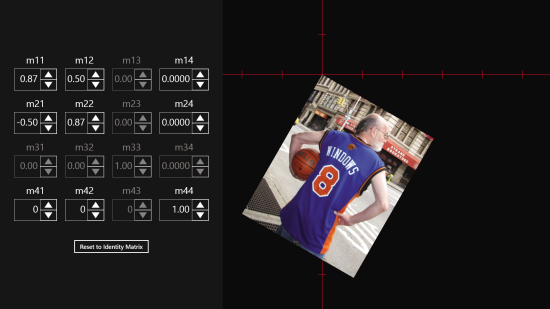Figure 2 The BitmapTransformExperiment Program

For your initial experimentations, restrict your attention to the top three rows and the leftmost three columns of the matrix. These make up a 3×3 matrix that performs the following transform:The 1×3 matrix at the left represents a 3D coordinate. For the bitmap, the x value ranges from 0 to the bitmap width; y ranges from 0 to the bitmap height; and z is 0.

When the 1×3 matrix is multiplied by the 3×3 transform matrix, the standard matrix multiplication results in transformed coordinates: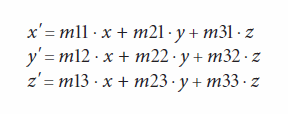The identity matrix—in which the diagonal elements of m11, m22, and m33 are all 1 and everything else is 0—results in no transform.

Because I’m starting out with a flat bitmap, the z coordinate is 0, hence m31, m32, and m33 have no effect on the result. When the transformed bitmap is rendered to the screen, the (x*', y', z') result is collapsed onto a flat 2D coordinate system by ignoring the z'* coordinate, which means that m13, m23, and m33 have no effect. This is why the third row and third column are grayed out in the BitmapTransformExperiment program. You can set values for these matrix elements, but they don’t affect how the bitmap is rendered.

What you’ll discover is that m11 is a horizontal scaling factor with a default value of 1. Make it larger or smaller to increase or decrease the bitmap width, or make it negative to flip the bitmap around the vertical axis. Similarly, m22 is a vertical scaling factor.

The m21 value is a vertical skewing factor: Values other than 0 turn the rectangular bitmap into a parallelogram as the right edge is shifted up or down. Similarly, m12 is a horizontal skewing factor.

A combination of horizontal skewing and vertical skewing can result in rotation. To rotate the bitmap clockwise by a particular angle, set m11 and m22 to the cosine of that angle, set m21 to the sine of the angle, and set m12 to the negative sine. Figure 2 shows rotation by 30 degrees.

In the context of 3D, the rotation shown in Figure 2 is actually rotation around the Z axis, which conceptually extends out from the screen. For an angle of α, the transform matrix looks like this:It’s also possible to rotate the bitmap around the Y axis or the X axis. Rotation around the Y axis doesn’t affect the y coordinate, so the transform matrix is this:If you try this with the BitmapTransformExperiment program, you’ll discover that only the m11 value has an effect. Setting it to the cosine of a rotation angle merely decreases the width of the rendered bitmap. That decrease in width is consistent with rotation around the Y axis.

Similarly, this is rotation around the X axis:In the BitmapTransformExperiment program, this results in reducing the height of the bitmap.

The signs of the two sine factors in the transform matrices govern the direction of rotation. Conceptually, the positive Z axis is assumed to extend from the screen, and the rotations follow the left-hand rule: Align the thumb of your left hand with the axis of rotation and point it toward positive values; the curve of your other fingers indicates the direction of rotation for positive angles.

The type of 3D transform represented by this 3×3 transform matrix is known as a linear transform. The transform only involves constants multiplied by the x, y and z coordinates. No matter what numbers you enter in the first three rows and columns of the transform matrix, the bitmap is never transformed into anything more exotic than a parallelogram; in three dimensions, a cube is always transformed into a parallelepiped.

You’ve seen how the bitmap can be scaled, skewed and rotated, but throughout this exercise, the upper-left corner of the bitmap has remained fixed at a single location. (That location is governed by a 2D transform set in the Render method prior to the DrawBitmap call.) The inability to move the upper-left corner of the bitmap results from the mathematics of the linear transform. There’s nothing in the transform formula that can shift a (0, 0, 0) point to another location, which is a type of transform known as translation.

## Achieving Translation

To understand how to obtain translation in 3D, let’s briefly think about 2D transforms.

In two dimensions, a linear transform is a 2×2 matrix, and it’s capable of scaling, skewing and rotating. To get translation as well, the 2D graphics are assumed to exist in 3D space but on a 2D plane where the z coordinate always equals 1. To accommodate the additional dimension, the 2D linear transform matrix is expanded into a 3×3 matrix, but usually the last row is fixed:The matrix multiplication results in these transform formulas:The m31 and m32 factors are the translation factors. The secret behind this process is that translation in two dimensions is equivalent to skewing in three dimensions.

An analogous process is used for 3D graphics: The 3D coordinate is actually assumed to exist in 4D space where the coordinate of the fourth dimension is 1. But to represent a 4D coordinate point, you have a silly little practical problem: A 3D point is (x, y, z) and no letter comes after z, so what letter do you use for the fourth dimension? The closest available letter is w, so the 4D coordinate is (x, y, z, w).

The 3D linear transform matrix is expanded to 4×4 to accommodate the extra dimension:The transform formulas are now:You now have translation along the X, Y, and Z axes with m41, m42, and m43. The calculation seems to occur in 4D space, but it’s actually restricted to a 3D cross-section of 4D space where the w coordinate is always 1.

## Homogenous Coordinates

If you play around with the m41 and m42 values in BitmapTransformExperiment, you’ll see they do indeed result in horizontal and vertical translation.

But what about that last row of the matrix? What happens if you don’t restrict that last row to 0s and 1s? Here’s the full 4×4 transform applied to a 3D point: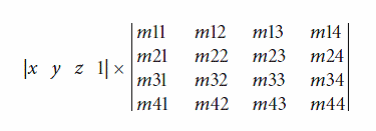The formulas for x*', y'* and z*'* remain the same, but w*'* is now calculated like this: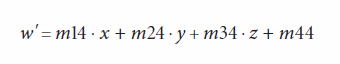And that’s a real problem. Previously, a little trick was employed to use a 3D cross-section of 4D space where the w coordinate always equals 1. But now the W coordinate is no longer 1, and you’ve been propelled out of that 3D cross-section. You’re lost in 4D space, and you need to get back to that 3D cross-section where w equals 1.

There’s no need to build a trans-dimensional space-time machine, however. Fortunately, you can make the leap mathematically by dividing all the transformed coordinates by w*'*:Now w*'* equals 1 and you’re back home!

But at what cost? You now have a division in the transform formulas, and it’s easy to see how that denominator might be 0 in some circumstances. That would result in infinite coordinates.

Well, maybe that’s a good thing.

When German mathematician August Ferdinand Möbius (1790–1868) invented the system I’ve just described (called “homogenous coordinates” or “projective coordinates”), one of his goals was to represent infinite coordinates using finite numbers.

The matrix with the 0s and 1s in the last row is called an affine transform, meaning that it doesn’t result in infinity, so a transform capable of infinity is called a non-affine transform.

In 3D graphics, non-affine transforms are extremely important, for this is how perspective is achieved. Everyone knows that in real life, objects further from your eye appear to be smaller. In 3D graphics, you obtain that effect with a denominator that isn’t a constant 1.

Try it out in BitmapTransformExperiment: If you make m14 a small positive number—and only small values are necessary for interesting results—then values of x and y are proportionally decreased as x gets larger. Make m14 a small negative number, and larger values of x and y are increased. Figure 3 shows that effect combined with a non-zero m12 value. The rendered bitmap is no longer a parallelogram, and the perspective suggests that the right edge has swung closer to your eyes.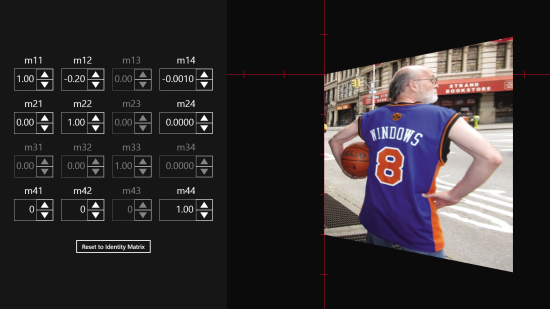Figure 3 Perspective in BitmapTransformExperiment

Similarly, non-zero values of m24 can make the top or bottom of the bitmap seemingly swing toward you or further away. In real 3D programming, it’s the m34 value that’s commonly used, for that allows objects to increase or decrease in size based on their z coordinates—their distance from the viewer’s eyes.

When a 3D transform is applied to 2D objects, the m44 value is usually left at 1, but it can function as an overall scaling factor. In real 3D programming, m44 is usually set to 0 when perspective is involved because conceptually the camera is at the origin. A 0 value of m44 only works if 3D objects don’t have z coordinates of 0, but when working with 2D objects, the z coordinate is always 0.

In applying this 4×4 transform matrix to a flat bitmap, you’re only making use of half the matrix elements. Even so, Figure 3 shows something you can’t do with the normal two-dimensional Direct2D transform matrix, which is to apply a transform that turns a rectangle into something other than a parallelogram. Indeed, the transform objects used in most of Direct2D are called D2D1_MATRIX_3X2_F and Matrix3x2F, emphasizing the inaccessibility of the third row and the inability to perform non-affine transforms.

With D2D1_MATRIX_4X4_F, it’s possible to derive a transform that maps a bitmap into any convex quadrilateral—that is, any arbitrary four-sided figure where the sides don’t cross and where interior angles at the vertices are less than 180 degrees.

If you don’t believe me, try playing around with the NonAffineStretch program. Note that this program is adapted from a Windows Runtime program, also called NonAffineStretch, in Chapter 10 of my book, “Programming Windows, 6th Edition” (Microsoft Press, 2013).

Figure 4 shows NonAffineStretch in use. You can use the mouse or your fingers to drag the green dots to any location on the screen. As long as you keep the figure a convex quadrilateral, a 4×4 transform can be derived based on the dot locations. That transform is used to draw the bitmap and is also displayed in the lower-right corner. Only eight values are involved; the elements in the third row and third column are always default values, and m44 is always 1.Figure 4 The NonAffineStretch Program

The mathematics behind this are a little hairy, but the derivation of the algorithm is shown in Chapter 10 of my book.

## The Matrix4x4F Class

To make working with the D2D1_MATRIX_4X4_F structure a bit easier, the Matrix4x4F class in the D2D1 namespace derives from that structure. This class defines a constructor and a multiplication operator (which I used in the NonAffineStretch algorithm), and several helpful static methods for creating common transform matrices. For example, the Matrix4x4F::RotationZ method accepts an argument that is an angle in degrees and returns a matrix that represents rotation by that angle around the Z axis: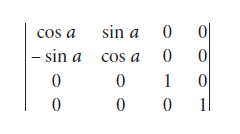Other Matrix4x4F functions create matrices for rotation around the X and Y axes, and rotation around an arbitrary axis, which is a much more difficult matrix.

A function called Matrix4x4F::PerspectiveProjection has an argument named depth. The matrix it returns is:This means the transform formula for x’ is: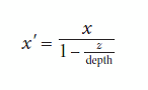And similarly for y*'* and z*'*, which means whenever the z coordinate equals depth, the denominator is 0, and all the coordinates become infinite.

Conceptually, this means you’re viewing the computer screen from a distance of depth units, where the units are the same as the screen itself, meaning pixels or device-independent units. If a graphical object has a z coordinate equal to depth, it’s depth units in front of the screen, which is right at your eyeball! That object should appear very large to you—mathematically infinite.

But wait a minute: The sole purpose of the D2D1_MATRIX_4X4_Fstructure and the Matrix4x4F class is for calls to DrawBitmap, and bitmaps always have z coordinates of 0. So how does this m34 value of –1/depth have any effect at all?

If the PerspectiveProjection matrix is used by itself in the DrawBitmap call, it will indeed have no effect. But it’s intended to be used in conjunction with other matrix transforms. Matrix transforms can be compounded by multiplying them. Although the original bitmap has no z coordinates, and z coordinates are ignored for rendering, z coordinates can certainly play a role in the compounding of transforms.

Let’s look at an example. The RotatingText program creates a bitmap with the text “ROTATE” with a width that’s just about half the width of the screen. Much of the Update and Render methods are shown in Figure 5.

Figure 5 Code from RotatingTextRenderer.cpp

``````void RotatingTextRenderer::Update(DX::StepTimer const& timer)
{
...
// Begin with the identity transform
m_matrix = Matrix4x4F();
// Rotate around the Y axis
double seconds = timer.GetTotalSeconds();
float angle = 360 * float(fmod(seconds, 7) / 7);
m_matrix = m_matrix * Matrix4x4F::RotationY(angle);
// Apply perspective based on the bitmap width
D2D1_SIZE_F bitmapSize = m_bitmap->GetSize();
m_matrix = m_matrix *
Matrix4x4F::PerspectiveProjection(bitmapSize.width);
}
void RotatingTextRenderer::Render()
{
...
ID2D1DeviceContext* context =
m_deviceResources->GetD2DDeviceContext();
Windows::Foundation::Size logicalSize =
m_deviceResources->GetLogicalSize();
context->SaveDrawingState(m_stateBlock.Get());
context->BeginDraw();
context->Clear(ColorF(ColorF::DarkMagenta));
// Move origin to top center of screen
Matrix3x2F centerTranslation =
Matrix3x2F::Translation(logicalSize.Width / 2, 0);
context->SetTransform(centerTranslation *
m_deviceResources->GetOrientationTransform2D());
// Draw the bitmap
context->DrawBitmap(m_bitmap.Get(),
nullptr,
1.0f,
D2D1_INTERPOLATION_MODE_LINEAR,
nullptr,
&m_matrix);
...
}
``````

In the Update method, the Matrix4x4F::RotationY method creates the following transform: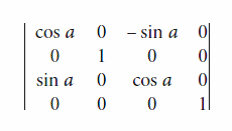Multiply this by the matrix shown earlier returned from the Matrix4x4F::PerspectiveProjection method, and you’ll get:The transform formulas are:These definitely involve perspective, and you can see the result in Figure 6.Figure 6 The RotatingText Display

Watch out: The depth argument to Matrix4x4F::Perspective­Projection is set to the bitmap width, so as the rotating bitmap swings around, it might come very close to your nose.

Charles Petzold is a longtime contributor to MSDN Magazine and the author of “Programming Windows, 6th Edition” (Microsoft Press, 2013), a book about writing applications for Windows 8. His Web site is charlespetzold.com.

Thanks to the following Microsoft technical experts for reviewing this article: Jim Galasyn and Mike Riches